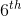# Common Core: 6th Grade Math : Recognize a Statistical Question as One that Anticipates Variability in the Data: CCSS.Math.Content.6.SP.A.1

## Example Questions

← Previous 1

### Example Question #1 : Statistics & Probability

Determine whether or not the following question can be considered as a statistical question.

Is Megan taller than Jim?

No

Yes

No

Explanation:

Statistical questions are characterized by possessing inherent variability in the data that is used to answer the question.

In this question, there is no variability because Megan is either taller than Jim or she is not; therefore, it is not a statistical question.

### Example Question #2 : Recognize A Statistical Question As One That Anticipates Variability In The Data: Ccss.Math.Content.6.Sp.A.1

Determine whether or not the following question can be considered as a statistical question.

Are the girls in thegrade taller than the boys in thegrade?

No

Yes

Yes

Explanation:

Statistical questions are characterized by possessing inherent variability in the data that is used to answer the question.

In this question, there is variability because the students are going to be different heights. Most likely, some girls will be taller than some boys, and some boys will be taller than some girls. To answer this question, we would have to find each height of the students in thegrade and then find the average height of the girls and the average height of the boys.

### Example Question #1 : Statistics & Probability

Determine whether or not the following question can be considered as a statistical question.

Are the cheetahs faster than lions?

No

Yes

Yes

Explanation:

Statistical questions are characterized by possessing inherent variability in the data that is used to answer the question.

In this question, there is variability because each animal will probably run at a different speed and their are many factors that could effect how fast a particular animal can run. For example, a younger lion might be able to run faster than an elderly cheetah. To answer this question, we would have to find averages of how fast a group of cheetahs run and how fast a group of lions run.

### Example Question #1 : Statistics & Probability

Determine whether or not the following question can be considered as a statistical question.

Can Melissa's dog run faster than Emily's dog?

Yes

No

No

Explanation:

Statistical questions are characterized by possessing inherent variability in the data that is used to answer the question.

In this question, there is no variability because Melissa's dog can either run faster than Emily's dog or it cannot; therefore, it is not a statistical question.

### Example Question #1 : Statistics & Probability

Determine whether or not the following question can be considered as a statistical question.

Did Joe score higher on the math test today than Melissa scored?

Yes

No

No

Explanation:

Statistical questions are characterized by possessing inherent variability in the data that is used to answer the question.

In this question, there is no variability because Joe is either scored higher on the test than Melissa or he did not; therefore, it is not a statistical question.

### Example Question #2 : Statistics & Probability

Determine whether or not the following question can be considered as a statistical question.

Do doctors make more money than lawyers?

No

Yes

Yes

Explanation:

Statistical questions are characterized by possessing inherent variability in the data that is used to answer the question.

In this question, there is variability because not all doctors and lawyers make the same amount. Some doctors might make more than some lawyers, and some lawyers might make more than some doctors. To answer this question, we would have to take a sample of doctors and lawyers to find out how much each person makes, then find the average income of each group.

### Example Question #1 : Recognize A Statistical Question As One That Anticipates Variability In The Data: Ccss.Math.Content.6.Sp.A.1

Determine whether or not the following question can be considered as a statistical question.

Does Andrew make more money than Laura?

No

Yes

No

Explanation:

Statistical questions are characterized by possessing inherent variability in the data that is used to answer the question.

In this question, there is no variability because Andrew either makes more money than Laura or he does not; therefore, it is not a statistical question.

### Example Question #8 : Recognize A Statistical Question As One That Anticipates Variability In The Data: Ccss.Math.Content.6.Sp.A.1

Determine whether or not the following question can be considered as a statistical question.

How old is Armen?

No

Yes

No

Explanation:

Statistical questions are characterized by possessing inherent variability in the data that is used to answer the question.

In this question, there is no variability because Armen is a certain age; therefore, it is not a statistical question.

### Example Question #2 : Statistics & Probability

Determine whether or not the following question can be considered as a statistical question.

How many boxes of cookies did each girl sell?

Yes

No

Yes

Explanation:

Statistical questions are characterized by possessing inherent variability in the data that is used to answer the question.

In this question, there is variability because each girl will probably sell a different amount of boxes. To answer this question, we would have to find out how many boxes of cookies each girl sold.

### Example Question #1 : Recognize A Statistical Question As One That Anticipates Variability In The Data: Ccss.Math.Content.6.Sp.A.1

Determine whether or not the following question can be considered as a statistical question.

What is the average test score of a class ofstudents?

Yes

No

Yes

Explanation:

Statistical questions are characterized by possessing inherent variability in the data that is used to answer the question.

In this question, there is variability because each student probably scored differently on the test. To answer this question, we would have to find each student's score and then find the average. Yes

← Previous 1

### All Common Core: 6th Grade Math Resources